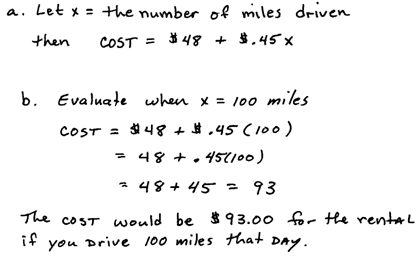## Pages

### Introduction to Algebra

The first step in understanding algebra is to realize that letters are used to represent numbers. Sometimes the problem will specifically state what number the letter represents, in which case, you replace the letter with that number and then simplify.Incorrect order of operations is the most common error when evaluating variable expressions.  To avoid this problem, particularly when beginning to learn algebra, we should put blank parentheses wherever we see a variable and then substitute in the appropriate values. Writing the parentheses may seem like unnecessary extra work but they will help us follow the correct order of operations and avoid many common errors.

Evaluate.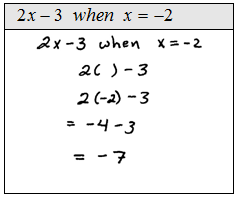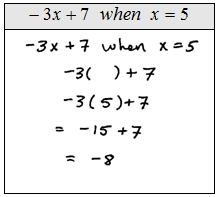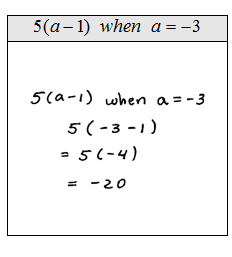When evaluating variable expressions with exponents be sure to take care with the order of operations. Remember that exponents should be evaluated before multiplication.Evaluate.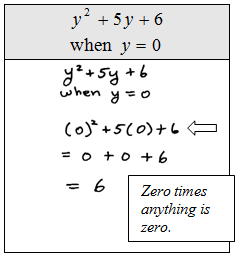Substitute the given value for each occurrence of the variable. Also it is best to perform the operations within the parentheses first rather than applying the distributive property.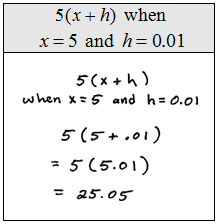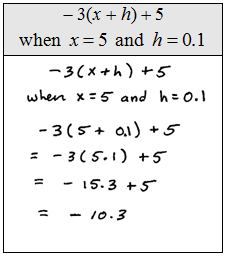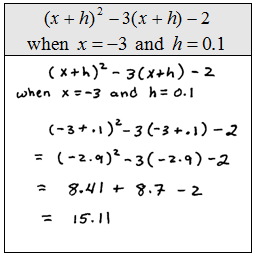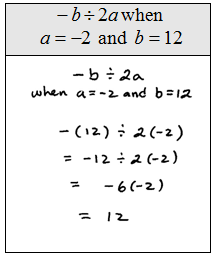Rental Truck -The daily truck rental rate is \$48.00 plus an additional \$0.45 for every mile driven.
a. Set up an algebraic equation for the daily cost of the truck.
b. Use it to determine the cost if someone plans to drive it 100 miles for the day.